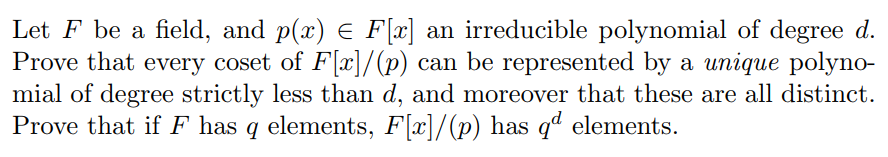# Let F be a field, and p(x) E F[x] an irreducible polynomial of degree od.Prove that every coset of Flr]/(p) can be represented by a unique polyno-mial of degree strictly less than d, and moreover that these are all distinct.Prove that if F has q elements, F[x]/(p) has q elements.has ql elements.

Question

Abstract Algebra. Please explain everything in detail.help_outlineImage TranscriptioncloseLet F be a field, and p(x) E F[x] an irreducible polynomial of degree od. Prove that every coset of Flr]/(p) can be represented by a unique polyno- mial of degree strictly less than d, and moreover that these are all distinct. Prove that if F has q elements, F[x]/(p) has q elements. has ql elements. fullscreen
check_circleExpert Solution
Step 1

To prove the statements regarding the quotient ring F[x]/(p(x)), under the given conditions

Step 2

The first part of the problem (regarding the degree and the uniqueness of the coset representative) is a consequence of the fact that F[x] is a Euclidean domain with degree as the norm.

Step 3

We have already proved that every coset can be represented by a polynomial b(x) of degree less than the degree of p)(x). Here is the proof of the uniqueness of b(x) (for each coset). The main point is that for ...

### Want to see the full answer?

See Solution

#### Want to see this answer and more?

Solutions are written by subject experts who are available 24/7. Questions are typically answered within 1 hour*

See Solution
*Response times may vary by subject and question
Tagged in

### Math